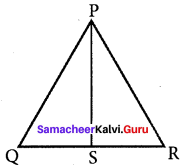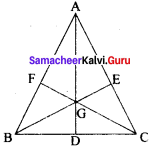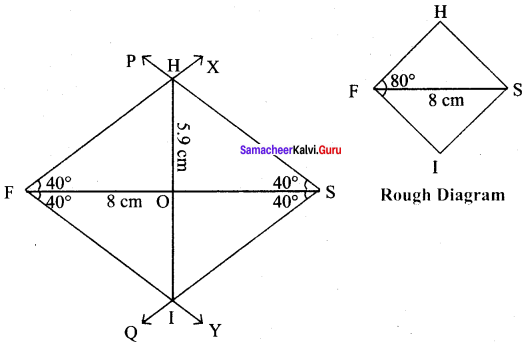Students can Download Maths Chapter 3 Geometry Additional Questions and Answers, Notes Pdf, Samacheer Kalvi 8th Maths Book Solutions Guide Pdf helps you to revise the complete Tamilnadu State Board New Syllabus and score more marks in your examinations.

## Tamilnadu Samacheer Kalvi 8th Maths Solutions Term 3 Chapter 3 Geometry Additional Questions

Question 1.
In ΔPQR, PS is a median. If QS = 7 cm find the length of QR?
Solution:
PS is the median ⇒ S is the midpoint of QRGiven
QS = 7 cm
∴ SR = 7 cm
QR = QS + SR
= 7 + 7 = 14cm

Question 2.
In ΔABC, G is the centroid. If AD = 6 cm, BC = 4 cm and BE = 9 cm find the perimeter of ΔBDG.
Solution:
In ΔABC, G is the centroid.⇒ GD = $$\frac{1}{3}$$ of AD = $$\frac{1}{3}$$ (6)
BE = 9 cm
⇒ BG = $$\frac{2}{3}$$ of BE = $$\frac{2}{3}$$ (9) = 6cm
Also D is the midpoint of BC ⇒ BD
= $$\frac{1}{1}$$ of BC = $$\frac{1}{2}$$ (4) = 2cm
∴ Perimeter of ΔBDG = BD + GD + BG = 2 + 2 + 6 = 10 cmQuestion 3.
Construct a rhombus FISH with FS = 8 cm and ∠F = 80°
Solution:
Given FS = 8 cm and ∠F = 80°Steps :
(i) Drawn a line segment FS = 7 cm.
(ii) At F, made ∠SFX = ∠SFY = 40° on either side of FS.
(iii) At S, made ∠FSP = ∠FSQ = 40° on either side of FS
(iv) Let FX and SP cut at H and FY and SQ cut at I.
(v) FISH is the required rhombus

Calculation of Area:
Area of the rhombus FISH = $$\frac{1}{2}$$ × d1 × d2 sq.units = $$\frac{1}{2}$$ × 5.9 × 7 cm² = 20.65 cm²